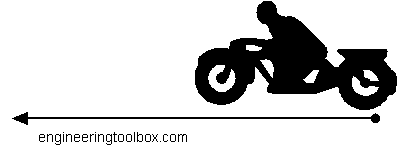Engineering ToolBox - Resources, Tools and Basic Information for Engineering and Design of Technical Applications!

# Acceleration

## Change in velocity vs. time used.

Acceleration is the ratio change in velocity to time used and can be expressed as

a = dv / dt

= (v1 - v0) / (t1 - t0)      (1)

where

a = acceleration (m/s2, ft/s2)

dv = change in velocity (m/s, ft/s)

v1 = final speed (m/s, ft/s)

v0 = initial speed (m/s, ft/s)

dt = time taken (s)

t1 = final time (s)

t0 = initial time (s)

### Example - Motorcycle AccelerationIf a motorcycle accelerates from 0 km/h to 100 km/h in 3 seconds, the acceleration can be calculated as

a = ((100 km/h) - (0 km/h)) (1000 m/km) / (3600 s/h)) / (3 s)

= 9.26 (m/s2)

or close to acceleration of gravity 1 g = 9.81 (m/s2)

The time from 0 - 100 km/h for an acceleration equal to gravity can be calculated by modifying the equation above to

(9.81 m/s2) = ((100 km/h) - (0 km/h)) (1000 m/km) / (3600 s/h)) / dt

or

dt = ((100 km/h) - (0 km/h)) (1000 m/km) / (3600 s/h)) / (9.81 m/s2)

= 2.83 s

### Acceleration Calculator

The calculator below can be used to calculate acceleration:

v0 - initial velocity

v1 - final velocity

km/h

m/s

mph

ft/s

time taken (s)

## Related Topics

• ### Dynamics

Motion - velocity and acceleration, forces and torque.

## Related Documents

• ### Acceleration of Gravity and Newton's Second Law

Acceleration of gravity and Newton's Second Law - SI and Imperial units.
• ### Acceleration Units Converter

Converting between units of acceleration.
• ### Acceleration vs. Velocity Equations

Useful equations related to acceleration, average velocity, final velocity and distance traveled.
• ### Capacitors

Capacitors and capacitance - charge and unit of charge.
• ### Car - Traction Force

Adhesion and tractive force between car wheel and surface.
• ### Car Acceleration

Car acceleration calculator.
• ### Centripetal and Centrifugal Acceleration Force

Forces due to circular motion and centripetal / centrifugal acceleration.
• ### Conn-Rod Mechanism

The connecting rod mechanism.
• ### Conservation of Momentum

The momentum of a body is the product of its mass and velocity - recoil calculator.
• ### Disk Brakes - Torque and Force

Forces and torque activated with disk brakes.
• ### Force

Newton's third law - force vs. mass and acceleration.
• ### Formulas of Motion - Linear and Circular

Linear and angular (rotation) acceleration, velocity, speed and distance.
• ### Mass vs. Weight

Mass vs. weight - the Gravity Force.
• ### Piston Engines - Displacement

Calculate piston engine displacement.
• ### Rolling Resistance

Rolling friction and rolling resistance.

## Engineering ToolBox - SketchUp Extension - Online 3D modeling!

Add standard and customized parametric components - like flange beams, lumbers, piping, stairs and more - to your Sketchup model with the Engineering ToolBox - SketchUp Extension - enabled for use with older versions of the amazing SketchUp Make and the newer "up to date" SketchUp Pro . Add the Engineering ToolBox extension to your SketchUp Make/Pro from the Extension Warehouse !

We don't collect information from our users. More about

## Citation

• The Engineering ToolBox (2008). Acceleration. [online] Available at: https://www.engineeringtoolbox.com/acceleration-d_1393.html [Accessed Day Month Year].

Modify the access date according your visit.

9.19.12